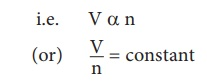Home | | Science 10th Std | Fundamental Laws of Gases

# Fundamental Laws of Gases

The three fundamental laws which connect the relation between pressure, volume and temperature are as follows: 1. Boyle’s Law 2. Charles's law 3. Avogadro's law

FUNDAMENTAL LAWS OF GASES

The three fundamental laws which connect the relation between pressure, volume and temperature are as follows:

1.              Boyle’s Law

2.              Charles's law

## 1. Boyle’s law:

When the temperature of a gas is kept constant, the volume of a fixed mass of gas is inversely proportional to its pressure. This is shown in Figure 3.6.

P α 1/VIn other words, for an invariable mass of a perfect gas, at constant temperature, the product of its pressure and volume is a constant.

(i.e) PV = constant

## 2. Charles's law(The law of volume)

Charles’s law was formulated by a French scientist Jacques Charles. According to this law, When the pressure of gas is kept constant, the volume of a gas is directly proportional to the temperature of the gas.

V α T

or V/T = constantAvogadro's law states that at constant pressure and temperature, the volume of a gas is directly proportional to number of atoms or molecules present in it.

i.e. V α n

(or) V/n = constantAvogadro’s number (NA) is the total number of atoms per mole of the substance. It is equal to 6.023 × 1023 /mol.

Study Material, Lecturing Notes, Assignment, Reference, Wiki description explanation, brief detail
10th Science : Chapter 3 : Thermal Physics : Fundamental Laws of Gases |